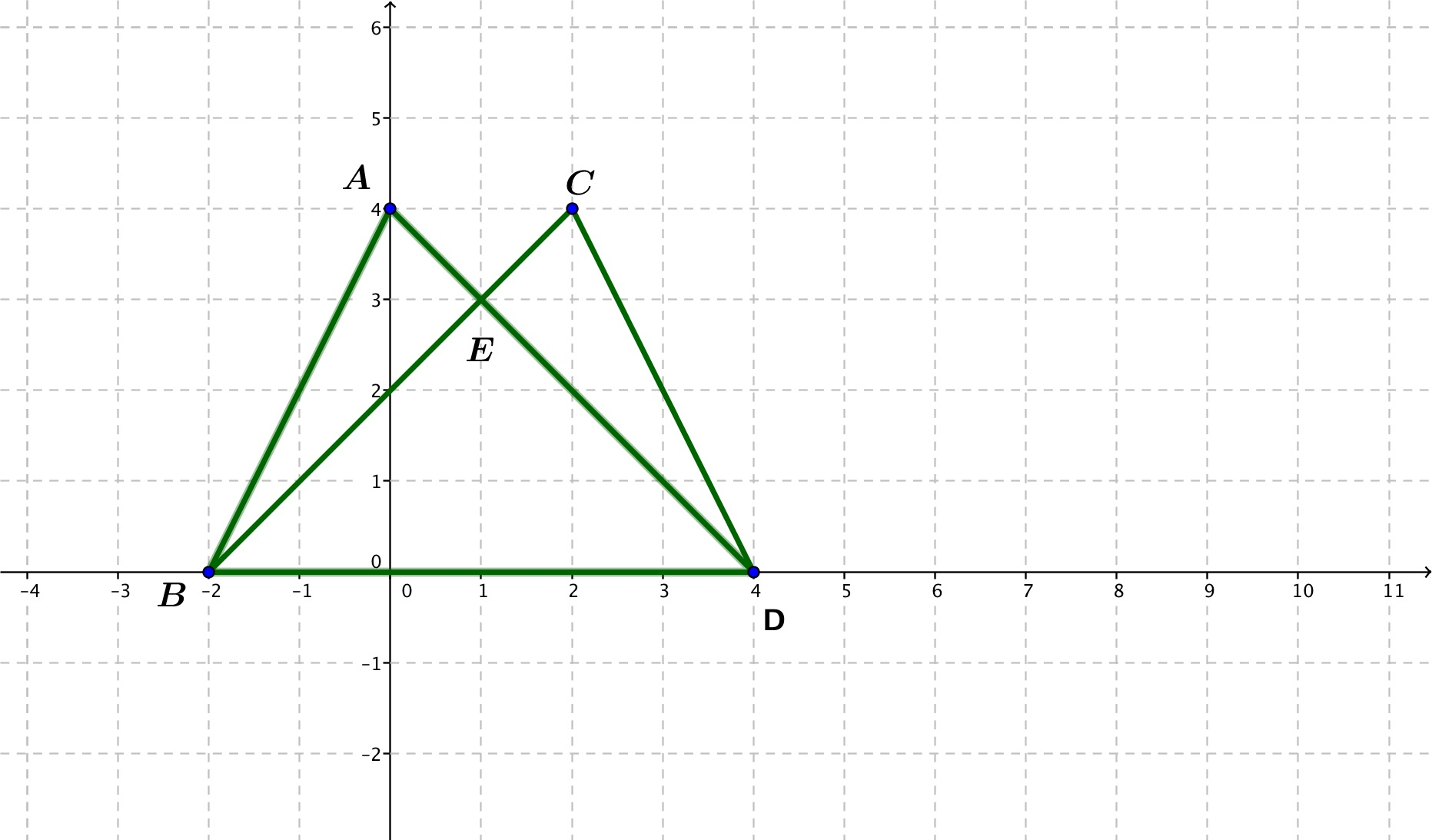# GEOM 3 | Lesson 1 | Practice 21. Consider the following two triangles:  $$ΔABD$$ and $$ΔCDB$$ are they congruent?

Why or why not?  Mark the congruent parts.1. Are ΔACD and ΔDBA congruent?  Why or why not?  Mark the congruent parts.  Remember that the opposite sides of a parallelogram are congruent and parallel.  Also recall that the alternate interior angles formed by parallel lines cut by a transversal are congruent.